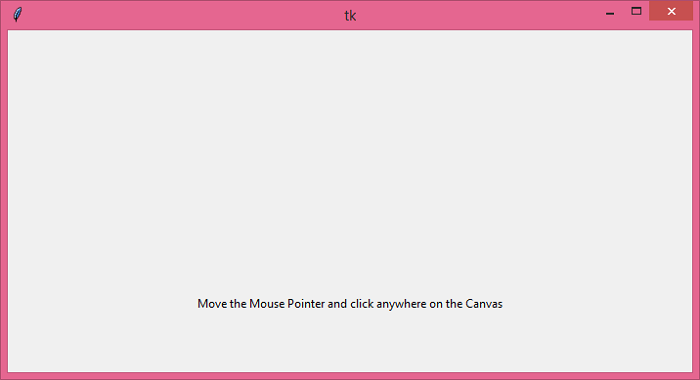# How to get coordinates on scrollable canvas in Tkinter?

The canvas widget has two coordinate systems: (a) Window coordinate system and (b) canvas coordinate system. The window coordinate system always starts from the leftmost corner (0,0) in the window, while the canvas coordinate system specifies where the items are actually placed in the canvas.

To convert the window coordinate system to canvas coordinate system, we can use the following two methods,

canvasx(event.x)
canvas(event.y)

If we consider the case for the window coordinate system, then mouse events occur only in the window coordinate system. We can convert the window coordinate to a canvas coordinate system.

## Example

In this application, we will get the position of the mouse pointer inside a canvas widget.

# Import the required libraries
from tkinter import *

# Create an instance of tkinter frame or window
win = Tk()

# Set the size of the window
win.geometry("700x350")

# Create a canvas widget
canvas = Canvas(win)
canvas.pack()

def on_button_pressed(event):
start_x = canvas.canvasx(event.x)
start_y = canvas.canvasy(event.y)
print("start_x, start_y =", start_x, start_y)

def on_button_motion(event):
end_x = canvas.canvasx(event.x)
end_y = canvas.canvasy(event.y)
print("end_x, end_y=", end_x, end_y)

# Bind the canvas with Mouse buttons
canvas.bind("<Button-1>", on_button_pressed)
canvas.bind("<Button1-Motion>", on_button_motion)

# Add a Label widget in the window
Label(win, text="Move the Mouse Pointer and click " "anywhere on the Canvas").pack()

win.mainloop()

## Output

Running the above code will display a window.If we move the mouse pointer and click anywhere on the canvas, then it will print the relative coordinate of the pointer on the console.

start_x, start_y = 340.0 159.0